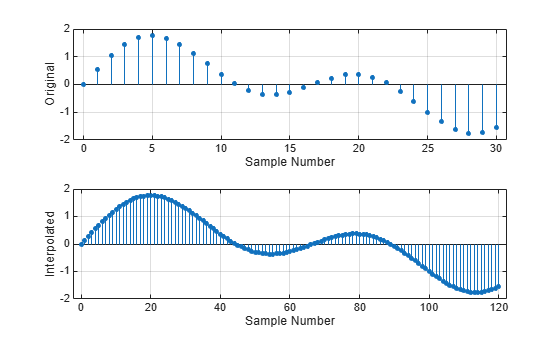# interp

Interpolation — increase sample rate by integer factor

## Syntax

``y = interp(x,r)``
``y = interp(x,r,n,cutoff)``
``[y,b] = interp(x,r,n,cutoff)``

## Description

example

````y = interp(x,r)` increases the sample rate of `x`, the input signal, by a factor of `r`.```
````y = interp(x,r,n,cutoff)` specifies two additional values: `n` is half the number of original sample values used to interpolate the expanded signal.`cutoff` is the normalized cutoff frequency of the input signal, specified as a fraction of the Nyquist frequency. ```
````[y,b] = interp(x,r,n,cutoff)` also returns a vector, `b`, with the filter coefficients used for the interpolation.```

## Examples

collapse all

Create a sinusoidal signal sampled at 1 kHz. Interpolate it by a factor of four.

```t = 0:1/1e3:1; x = sin(2*pi*30*t) + sin(2*pi*60*t); y = interp(x,4);```

Plot the original and interpolated signals.

```subplot(2,1,1) stem(0:30,x(1:31),'filled','MarkerSize',3) grid on xlabel('Sample Number') ylabel('Original') subplot(2,1,2) stem(0:120,y(1:121),'filled','MarkerSize',3) grid on xlabel('Sample Number') ylabel('Interpolated')```## Input Arguments

collapse all

Input signal, specified as a vector.

Data Types: `double` | `single`

Interpolation factor, specified as a positive integer.

Data Types: `double` | `single`

Half the number of input samples used for interpolation, specified as a positive integer. For best results, use `n` no larger than 10. The lowpass interpolation filter has length 2 × `n` × `r` + 1.

Data Types: `double` | `single`

Normalized cutoff frequency of the input signal, specified as a positive real scalar not greater than 1 that represents a fraction of the Nyquist frequency. A value of 1 means that the signal occupies the full Nyquist interval.

Data Types: `double` | `single`

## Output Arguments

collapse all

Interpolated signal, returned as a vector. `y` is `r` times as long as the original input, `x`.

Data Types: `double` | `single`

Lowpass interpolation filter coefficients, returned as a column vector.

Data Types: `double` | `single`

## Algorithms

Interpolation increases the original sample rate of a sequence to a higher rate. It is the opposite of decimation. `interp` inserts zeros into the original signal and then applies a lowpass interpolating filter to the expanded sequence. The function uses the lowpass interpolation algorithm 8.1 described in :

1. Expand the input vector to the correct length by inserting 0s between the original data values.

2. Design a special symmetric FIR filter that allows the original data to pass through unchanged and interpolates to minimize the mean-square error between the interpolated points and their ideal values. The filter used by `interp` is the same as the filter returned by `intfilt`.

3. Apply the filter to the expanded input vector to produce the output.

 Digital Signal Processing Committee of the IEEE Acoustics, Speech, and Signal Processing Society, eds. Programs for Digital Signal Processing. New York: IEEE Press, 1979.

 Oetken, G., Thomas W. Parks, and H. W. Schüssler. “New results in the design of digital interpolators.” IEEE® Transactions on Acoustics, Speech, and Signal Processing. Vol. ASSP-23, No. 3, June 1975, pp. 301–309.